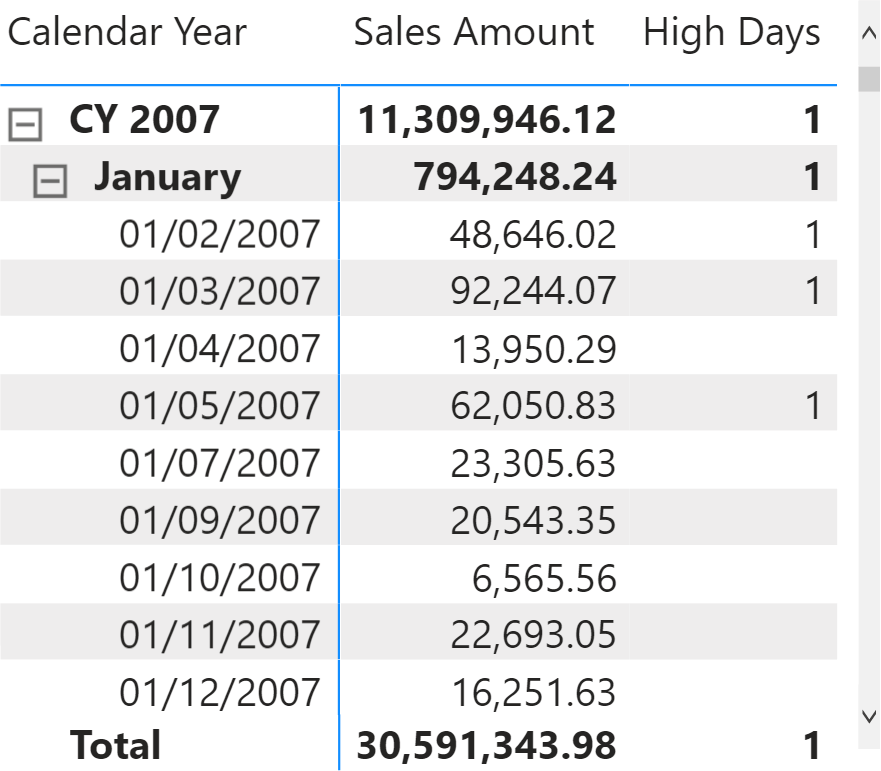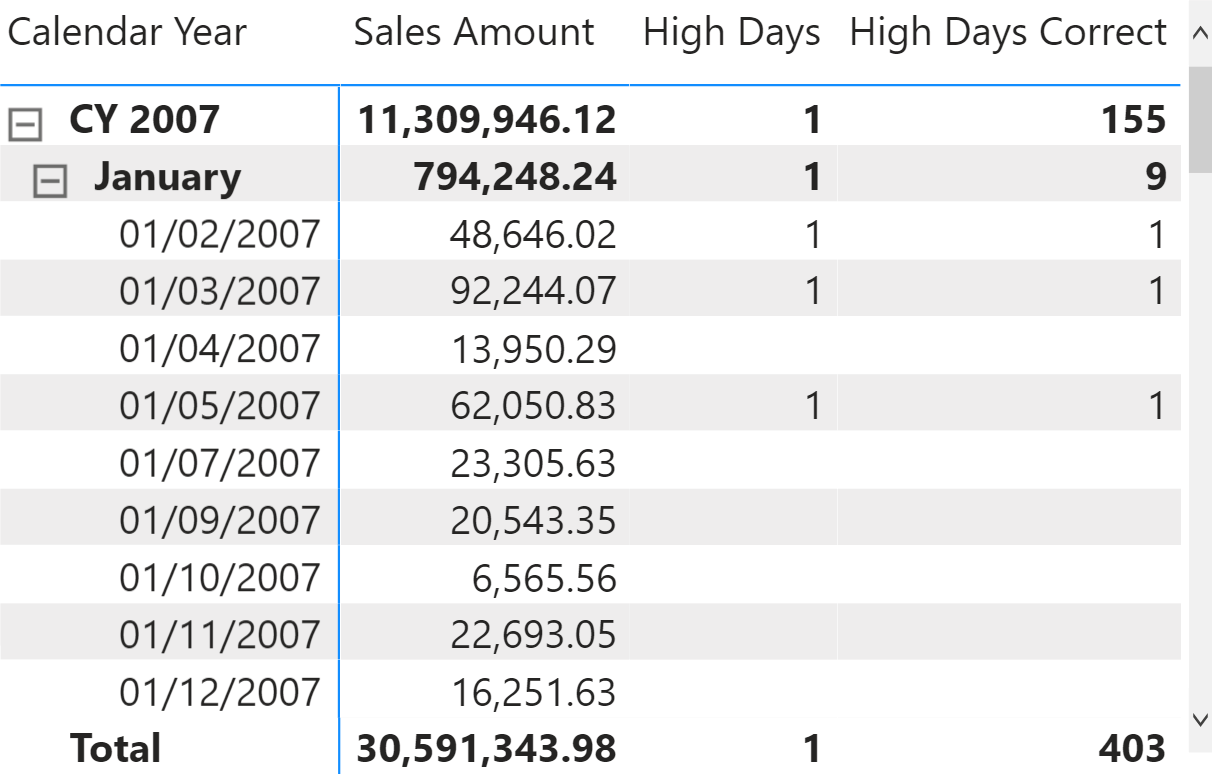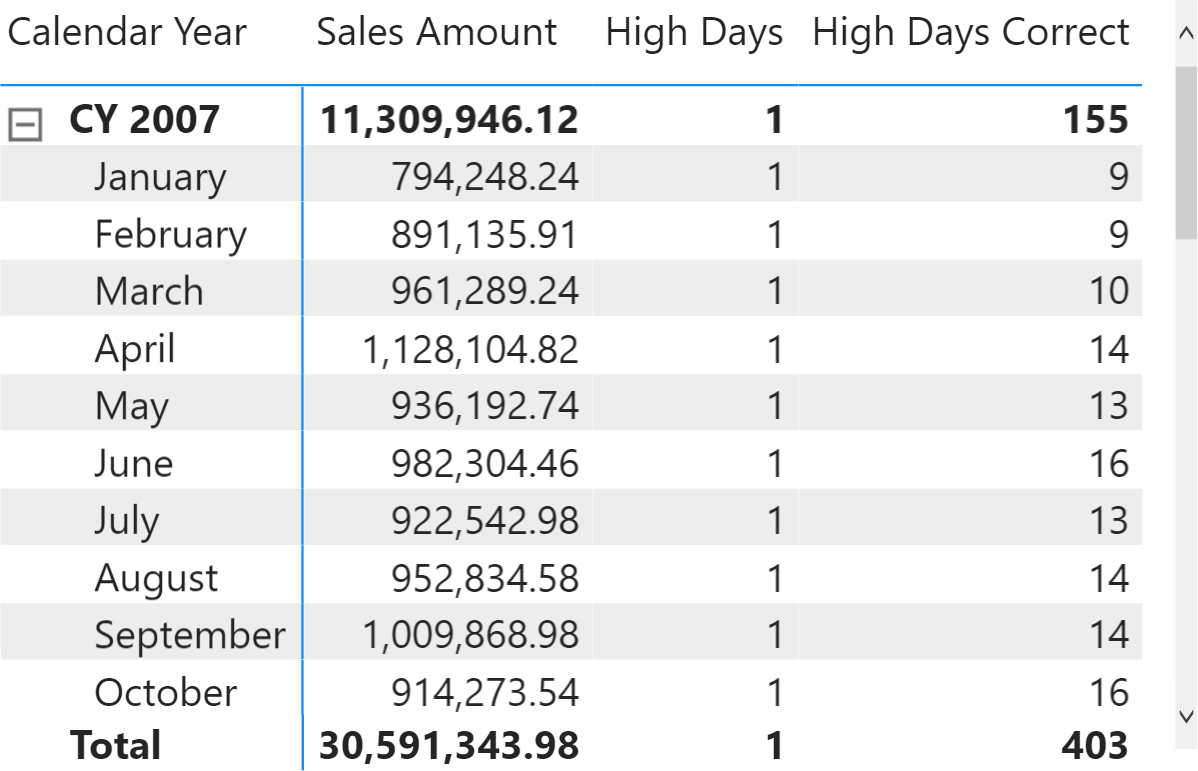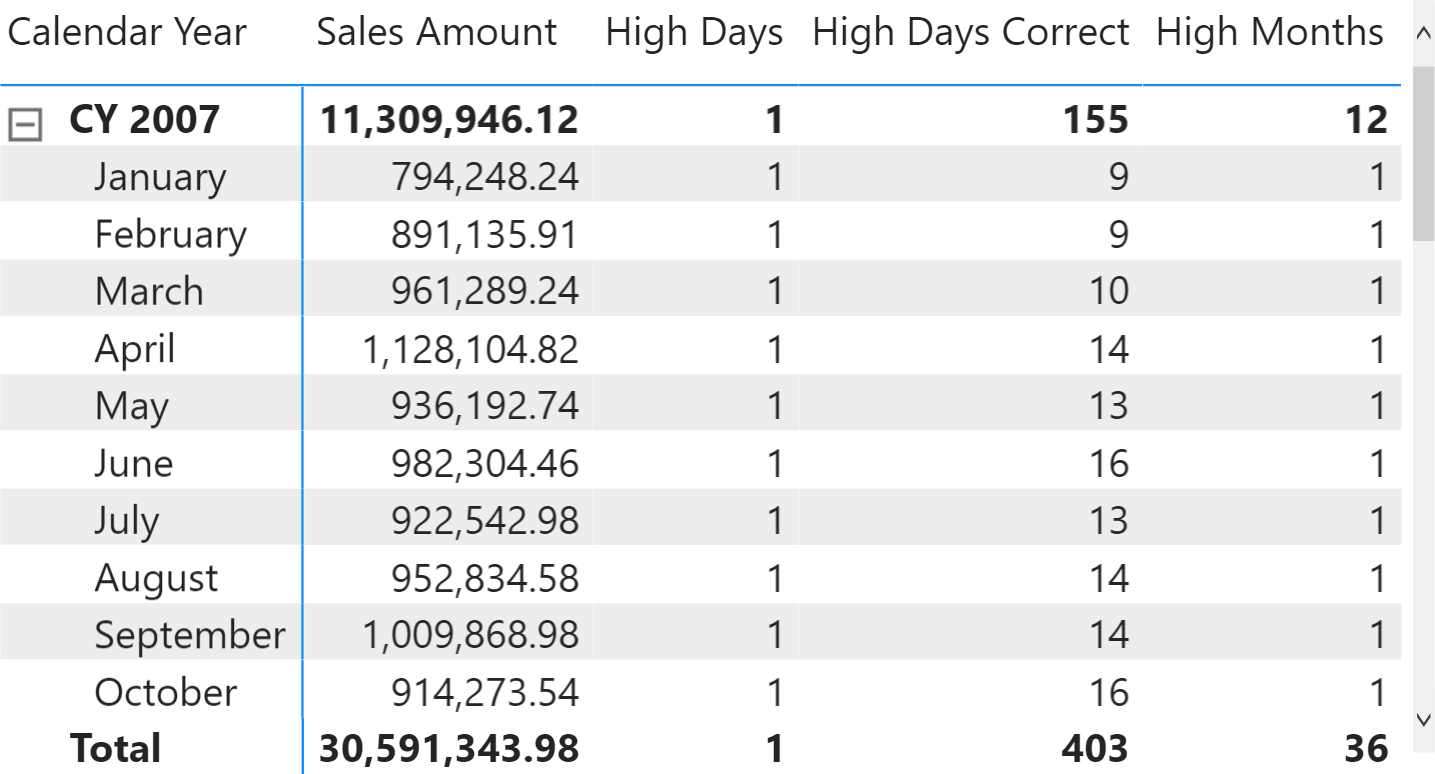In simple DAX measures, the total of a report is the sum of its individual rows. For more sophisticated measures, the total might seem wrong because the formula does not include an aggregation over the rows that are visible in the report. For example, if the total of a measure must be the sum of the values displayed in the rows of a report, we consider the expected result a “visual total”, which is a total that corresponds to the visual aggregation of its values sliced by different rows in the report.

Let us elaborate on the topic with a simple example: a measure counts on how many days inside a specific time period, Sales Amount is greater than 30,000. The measure below checks the condition and returns 1 if Sales Amount is greater than 30,000:

```High Days :=
IF ( [Sales Amount] > 30000, 1 )
```

The measure works fine in a report that shows one day in each row. However, the sub-totals and the total look wrong because they show 1 instead of the sum of the individual days.Be mindful that the total is correct, given the definition of the formula. Yet, it does not correspond to the “visual total” we would like to get. The reason is that the measure is evaluated for each cell of the report. The Total row does not correspond to the day level; it is a row that groups all the days into a single calculation. When DAX shows a value that looks wrong, it is not DAX that is not working. DAX is answering a question, perhaps the wrong one! Let us elaborate on this.

The measure checks whether the sales amount is greater than 30,000. It returns 1 if that is the case, and 0 otherwise. Because Sales Amount is greater than 30,000 in the Total row, the formula returns 1 as requested. The same happens in January. DAX answers the wrong question correctly!

The feeling that the total is wrong comes from the visual perception that we – as humans – obtain from the report. Let us look at the same report without the details at the day level.The results are identical, and we still have the feeling that the yearly total is wrong. The perception is now that the Total should be the sum of the individual months. Not seeing the day rows, we no longer expect the Total to be the sum of individual days.

DAX does not see the report the way we do. Therefore, we need to rephrase the question to obtain the desired result: We modify the formula granularity, which is the level of detail the formula needs to analyze.

The first question to ask yourself is: at what granularity should I check the total? It might be at the day level, the month level, or whatever time period. Let us say we set the granularity at the day level. The question becomes: at the day level, I want to check if the total is greater than 30,000. At any level above the day level, I want the sum of the values computed at the day level. Asking the question this way gets us closer to the correct formulation, but it is not perfect just yet.

Before getting to the correct formula, one further step is needed. The above description is bottom-up; we started at the detail level, and then the next step was to provide the aggregated result. In DAX, you need to reason top-down: first the aggregation, then the details. In other words, the same question should be stated as: for each day in the current time period, sum the result of this formula: if the sales of the day are greater than 30,000 then return 1, otherwise return 0. The question is the same. This time, we first provide the aggregation, and then we specify the calculation to perform at the detail level.

All this reasoning leads to the right formula:

```High Days Correct :=
SUMX (
'Date',
IF ( [Sales Amount] > 30000, 1 )
)
```

The formula iterates the Date table and computes the result for each day. Finally, it aggregates the daily results by using SUM.It is worth noting that there is no need to specify a different formula to compute a value at the day and the aggregated levels. Indeed, when a single day is selected, the formula behaves correctly: it only performs one iteration. Over any longer period of time, the number of iterations is the number of days in the period. Moreover, because the formula contains the desired granularity, it works no matter the granularity of the report. For example, the following report shows the right data at the month and the year levels, although the individual days are not visible.Once the question is phrased the right way, DAX behaves nicely. The granularity of the calculation depends on the definition of the formula. Each business and user might have different requirements. For example, to identify months with sales greater than 30,000, the granularity must be the month: for each month in the current period, sum the result of this formula: if the sales of that month are greater than 30,000 then return 1, otherwise return 0. The formula requires the same structure as the previous version; it just replaces the day with the month to perform the calculation at the right granularity.

The formula flows logically:

```High Months =
SUMX (
VALUES ( 'Date'[Calendar Year Month] ),
IF ( [Sales Amount] > 30000, 1 )
)
```

Indeed, when used in the report at the month level, the results are correct.The topic of granularity is not yet complete. There is still an issue with the formula above: the problem appears below the granularity supported by the formula. Indeed, the formula works at the month level – but what happens if we expand the month at the day level, showing details where the formula is not designed to work?The result is correct at the month level, but the High Months measure also returns values at the day level. The scenario is similar to the previous one: when the formula works at a granularity that it is not designed for, the result is counterintuitive. Fixing this issue requires other deep reasonings about what to do at a higher level of detail (aka granularity). We save this interesting topic for a new article in the coming weeks.

For now, here is our advice: if the total looks wrong, it might be because the DAX formula does not perform the calculation at the right granularity. Think about the business problem, define the right question, and implement the correct DAX expression.